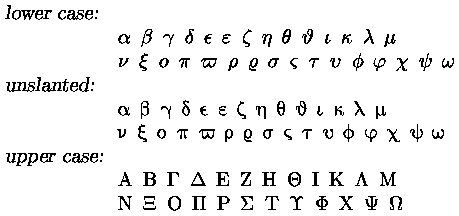Greek characters

There is no straighforward way to get Greek characters in HTML.
The way I do it is to use (La)TeX to get a PostScript file and convert to a transparent gif file. The (La)TeX commands for Greek characters are the following; the characters marked with an asterisk (*) also come in a "var"-version.

Greek
character
(La)TeX commands    Greek
character
(La)TeX commands
lower case upper case    lower case upper case
alpha \alpha A    nu \nu N
beta \beta B    xi \xi \Xi
gamma \gamma \Gamma    omicron o O
delta \delta \Delta    pi * \pi \Pi
epsilon * \epsilon E    rho * \rho P
zeta \zeta Z    sigma * \sigma \Sigma
eta \eta H    tau \tau T
theta * \theta \Theta    upsilon \upsilon \Upsilon
iota \iota I    phi * \phi \Phi
kappa \kappa K    chi \chi X
lambda \lambda \Lambda    psi \psi \Psi
mu \mu M    omega \omega \Omega

To get the right Greek characters, the macros and the 'o' of the lower case and the macros of the upper case have to given in math mode (i.e. between \$-signs). By default this gives slanted lower case characters. With a special trick these lower case letters can appear unslanted -- it is this unslanted version that I use on the page, as it looks better readable than the slanted version.
The full character set, including the "var"-versions, looks like this:Note:
The "X" in "(La)TeX" is pronounced as the Greek "chi" and as "ch" in the Scottish word "loch" -- not as an "x" !